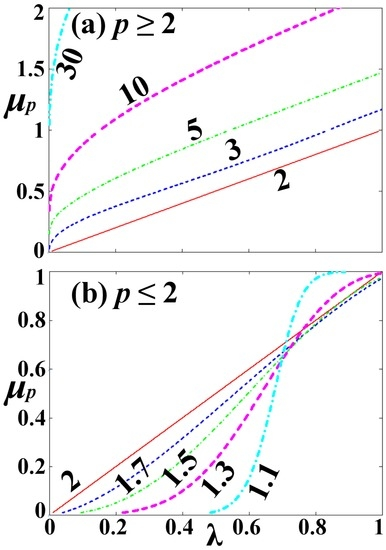Next Article in Journal
Calculating Iso-Committor Surfaces as Optimal Reaction Coordinates with Milestoning
Previous Article in Journal
Cauchy Principal Value Contour Integral with Applications
Open AccessArticle

# On the Convergence and Law of Large Numbers for the Non-Euclidean Lp -Means

Space Science and Engineering, Southwest Research Institute, San Antonio, TX 78238, USA
Academic Editors: Antonio Maria Scarfone and Kevin Knuth
Entropy 2017, 19(5), 217; https://doi.org/10.3390/e19050217
Received: 18 January 2017 / Revised: 13 April 2017 / Accepted: 9 May 2017 / Published: 11 May 2017
This paper describes and proves two important theorems that compose the Law of Large Numbers for the non-Euclidean $L p$ -means, known to be true for the Euclidean $L 2$ -means: Let the $L p$ -mean estimator, which constitutes the specific functional that estimates the $L p$ -mean of N independent and identically distributed random variables; then, (i) the expectation value of the $L p$ -mean estimator equals the mean of the distributions of the random variables; and (ii) the limit $N → ∞$ of the $L p$ -mean estimator also equals the mean of the distributions. View Full-Text
Show FiguresFigure 1

MDPI and ACS Style

Livadiotis, G. On the Convergence and Law of Large Numbers for the Non-Euclidean Lp -Means. Entropy 2017, 19, 217.